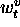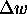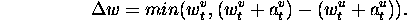### 4.1.1 Hydraulic erosion

Hydraulic erosion is caused by the presence of water in the form of rain. Water is dropped on each vertex in a high field and transfers the material. The basic method of transport is as follows: part of the material is dissolved in the water, the water runs down the hill and after the water evaporates the material holds in a new place. This approach can be easily extended from the height field representation to the set of triangles used in .

This physical model of hydraulic erosion is implemented with the assistance of height field (see Figure 8), where each vertex v at time t has altitude, amount of water, and volume of sedimentsuspended in the water. At each time step the excess of the water together with suspended sediment from vertex v is redistributed to the lower neighboring vertexes.Figure 8: One step of hydraulic erosion a)original b)c) two possibles results of erosion process

Let u denotes the neighboring vertex of v. The amount of redistributed wateris defined asIfis less than equal zero (the neighboring vertex u is greater or equal to vertex v) then the fragment of the sediment suspended in wateris deposited at vertex v. In other cases the excess of water is moved from higher vertex v to the lower vertex u and dissolved material is moved together with water.

Thus specified method simulates the relations necessary for the global process of erosion. The above algorithm are defined for two vertices. In full two dimensional implementation this has to be distributed to all lower neighbors.

Ivo Marak - marak@sgi.felk.cvut.cz